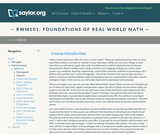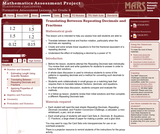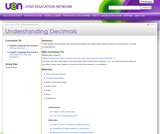Updating search results...

# 4 Results

View
Selected filters:
• FractionsUnrestricted Use
CC BY
Rating
0.0 stars

In this course, you will cover some of the most basic math applications, like decimals, percents, and even fractions. You will not only learn the theory behind these topics, but also how to apply these concepts to your life. You will learn some basic mathematical properties, such as the reflexive property, associative property, and others. The best part is that you most likely already know them, even if you did not know the proper mathematical terminology.

Material Type:
Activity/Lab
Full Course
Homework/Assignment
Syllabus
Provider:
The Saylor Foundation
08/28/2013Only Sharing Permitted
CC BY-NC-ND
Rating
4.0 stars

This lesson unit is intended to help teachers assess how well students are able to interpret percent increase and decrease, and in particular, to identify and help students who have the following difficulties: translating between percents, decimals, and fractions; representing percent increase and decrease as multiplication; and recognizing the relationship between increases and decreases.

Subject:
Ratios and Proportions
Material Type:
Assessment
Lesson Plan
Provider:
Shell Center for Mathematical Education
Provider Set:
Mathematics Assessment Project (MAP)
04/26/2013Only Sharing Permitted
CC BY-NC-ND
Rating
4.0 stars

This lesson unit is intended to help teachers assess how well students are able to: translate between decimal and fraction notation, particularly when the decimals are repeating; create and solve simple linear equations to find the fractional equivalent of a repeating decimal; and understand the effect of multiplying a decimal by a power of 10.

Subject:
Algebra
Ratios and Proportions
Material Type:
Assessment
Lesson Plan
Provider:
Shell Center for Mathematical Education
Provider Set:
Mathematics Assessment Project (MAP)
04/26/2013Educational Use
Rating
0.0 stars

These games and activities help students investigate the relationship between fractions and decimals, focusing on equivalence.

Subject:
Mathematics
Material Type:
Lesson Plan
Provider:
Utah Education Network